# Force

Force must be applied to an object to alter its speed or change its direction of movement. Force is calculated using the formula below: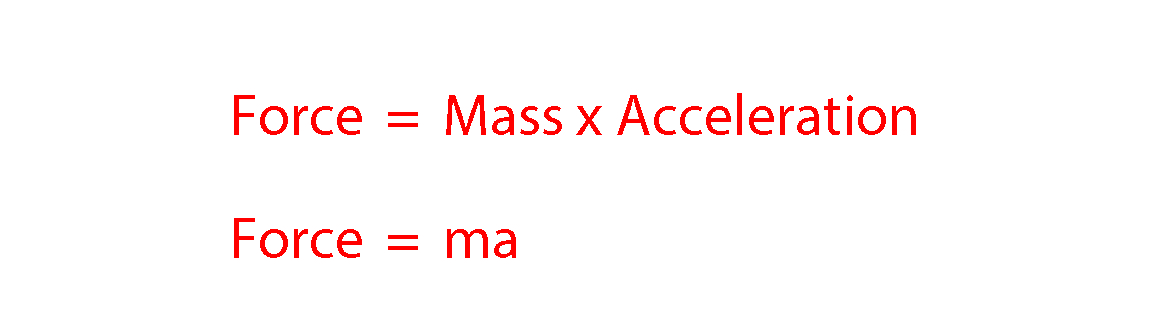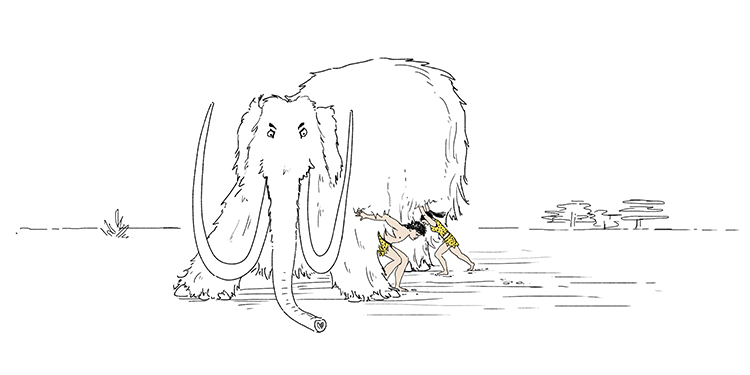Force the mammoth (mass)  away (acceleration).

## Force is measured in N (Newtons)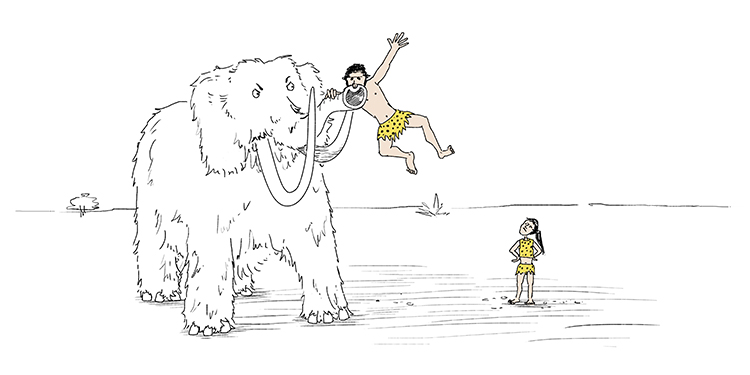Force the mammoth (mass) away (acceleration) but she just lifted me up by the nose (Newton).

NOTE:

This formula was famously discovered by Sir Isaac Newton. So important is this formula that the standard unit in which force is measured was named after Newton – Newtons (N).

Example 1

The diagram shows the horizontal forces acting on a car travelling along a straight road. The forward force is thrust and the rearward force is drag.

Calculate the size of the resultant force acting on the car.

If the car accelerates at 1.5m//s^2 what is its mass?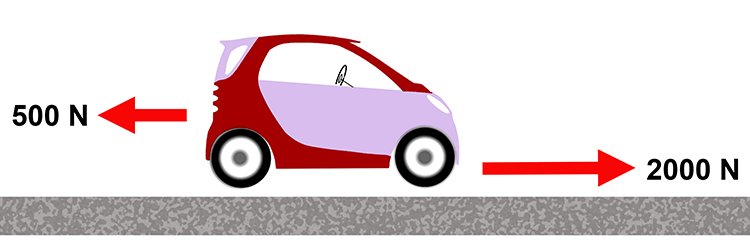Resultant force = 2000 – 500 = 1500N

Force = mass x acceleration

Divide both sides by acceleration to make mass the subject,

\frac{Force}{acceleration}=\frac{mass\times\strike{acceleration}}{\strike{acceleration}}

mass=\frac{Force}{acceleration}

Therefore the car's mass = 1500/1.5 = 1000kg

Example 2

A remote control car has a mass of 0.5kg. If its electric motor accelerates the car at 5m//s^2, what force does the electric motor provide?

Force = mass x acceleration

Force = 0.5 xx 5 = 2.5N

Example 3

The diagram shows the horizontal forces acting on a car of mass 1200 kg.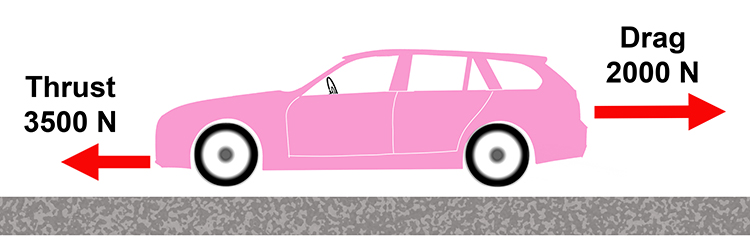Calculate the acceleration of the car at the instant shown in the diagram. Write down the equation you use, and then show clearly how you work out your answer and give the unit.